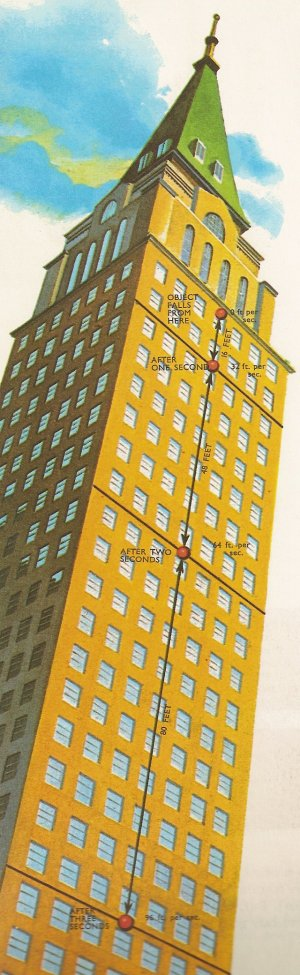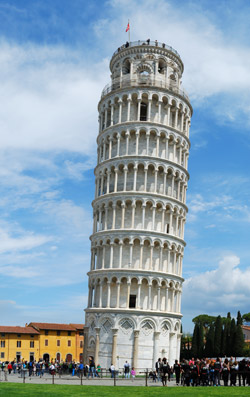A# acceleration due to gravity (g)Fig 1. An object that is allowed to fall freely will, if the effects of air resistance are ignored, gather speed (accelerate) at a rate of about 9.8 m/s2 (32 ft/s2). If dropped from rest, it will have fallen 4.9 m (16 ft) and be traveling at a speed of 9.8 m/s (32 ft/s) after 1 sec. After 2 sec, it will have fallen a further 14.7 m (48 ft) and be traveling at 19.6 m/s (64 ft/s). After 3 sec, it will have fallen a further 24.5 m (80 ft) and be traveling at 29.4 m/s (96 ft/s).

The acceleration due to gravity is the acceleration that an object experiences because of gravity when it falls freely close to the surface of a massive body, such as a planet. Also known as the acceleration of free fall, its value can be calculated from the formula

g = GM / (R + h) 2

where M is the mass of the gravitating body (such as the Earth), R is the radius of the body, h is the height above the surface, and G is the gravitational constant (= 6.6742 × 10–11 N·m2/kg2). If the falling object is at, or very nearly at, the surface of the gravitating body, then the above equation reduces to

g = GM / R 2

In the case of the Earth, g comes out to be approximately 9.8 m/s2 (32 ft/s2), though the exact value depends on location because of two main factors: Earth's rotation and Earth's equatorial bulge.

## Why g varies from place to place

The downward force of gravity is opposed by an outward centrifugal force due to the planet's rotation, which is greater at the equator than at higher latitudes. (The centrifugal force is "fictitious" in the sense that the real force caused by rotation is the centripetal force; however, it is a convenient fiction for the sake of calculations.) By itself, this effect would result in a range of values of g from 9.789 m/s2 (32.116 ft/s2) at the equator to 9.823 m/s2 (32.228 ft/s2) at the poles. This discrepancy is further accentuated because of Earth's equatorial bulge, which causes objects at lower latitudes to be further from the planet's center than objects nearer the poles and hence subject to a slightly weaker gravitational pull.

Overall these two effects result in a variation of 0.052 m/s2 (0.171 ft/s2) in the value of g, which leads to a variation in the weight of an object by about 0.5% depending on whether it is weighed at the equator or at one of the poles. Taking an average over the whole surface of the Earth, physicists have arrived at a standard value for g of 9.80665 m/s2 (32.1740 ft/s2). On other planets and moons the values of the acceleration due to gravity may be very different, resulting in different weights for the same object on these various worlds.

## Effect of air resistance

If Earth had no atmosphere, an object dropped from a great height would keep accelerating at a rate of 9.8 m/s2 (32 ft/s2) until it hit the ground. For example, if a person fell from an aircraft at an altitude of 10,000 m (32,808 ft), they would be traveling at about 442 m/s (1,450 ft/s) by the time they landed. In practice, this doesn't happen because of air resistance. The faster an object falls, the greater is the air resistance acting on it. At a certain velocity, known as the terminal velocity, the downward force of gravity is exactly balanced by the upward force of air resistance and there is no further acceleration.

If there were no atmosphere, all objects would fall at the same rate. This happens, for example, on the Moon. In one of the most memorable moments of the space program, David Scott, commander of the Apollo 15 mission, standing on the Moon's surface, dropped two objects – a geological hammer and a falcon's feather (the Apollo 15 lunar module was called Falcon) – at the same time from the same height. The feather didn't drift down, meanderingly, as it would have done on Earth. Instead, in the airless vacuum of space, it fell straight, without a flutter, keeping pace with the hammer and reaching the lunar surface at the same instant.

## Galileo and the Leaning Tower

One of the most famous stories in science is about Galileo Galilei and the Leaning Tower of Pisa. Galileo supposedly reached out from an upper balcony and let fall two stones of different weights. A remarkable thing happened: to the gasps and amazement of the crowd below, the stones hit the ground together. Although doubtless in part apocryphal, the account does at least have some backing from Galileo's pupil and amanuensis Viviani, who reported that Galileo had done the experiment "in front of all the faculty and students assembled."Leaning Tower of Pisa.

## g-force

Units of g, or 'gee', are used in aerospace when describing loads on aircraft or spacecraft. Someone who carried out remarkable experiments (on himself) involving very high g-forces was the pioneering aerospace physician John Stapp.